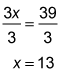##### Basic Math & Pre-Algebra Workbook For Dummies with Online Practice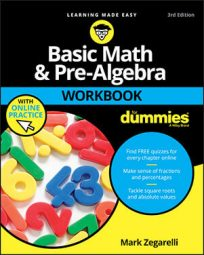You can solve algebraic equations by isolating x—that is, by getting x alone on one side of the equation and everything else on the other side. For most basic equations, isolating x involves a few steps, as you'll see in the following practice questions.

## Practice questions

1. Find the value of x in the equation 9x – 2 = 6x + 7.
2. Find the value of x in 4x – 17 = x + 22.

1. x = 3First, add or subtract the same number from each side to get all constants (non-x terms) on one side of the equation. In this example, you want to get all constants on the right side of the equation, so you add 2 to both sides: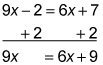Second, you add or subtract to get all x terms on the other side of the equation. Here, you want to get all x terms on the left side, so you subtract 6x from both sides: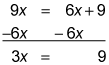Third, you divide by 3 to isolate x: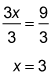2. x = 13Start by adding or subtracting the same number from each side to get all constants (non-x terms) on one side of the equation. Here, you add 17 to both sides to get all constants on the right side of the equation: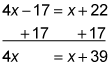The second step is to add or subtract to get all x terms on the other side of the equation. In this example, you need to subtract x from both sides to get all x terms on the left side: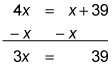The third step is to divide (in this case, by 3) to isolate x: